# Polar

(diff) ← Older revision | Latest revision (diff) | Newer revision → (diff)

The polar of a pointwith respect to a non-degenerate conic is the line containing all points harmonically conjugate towith respect to the points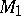and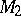of intersection of the conic with secants through(cf. Cross ratio). The pointis called the pole. If the pointlies outside the conic, then the polar passes through the points of contact of the two tangent lines that can be drawn through(see Fig. a). If the pointlies on the curve, then the polar is the tangent to the curve at this point. If the polar of the pointpasses through a point, then the polar ofpasses through(see Fig. b).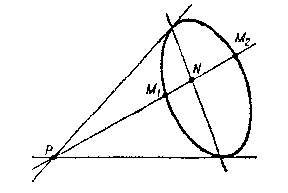Figure: p073400a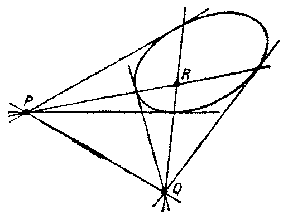Figure: p073400b

Every non-degenerate conic determines a bijection between the set of points of the projective plane and the set of its straight lines, which is a polarity (a polar transformation). Figures that correspond under this transformation are called mutually polar. A figure coinciding with its polar figure is called autopolar, or self-polar (see, for example, the self-polar triangle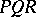in Fig. b).

Analogously one defines the polar (polar plane) of a point with respect to a non-degenerate surface of the second order.

The concept of a polar relative to a conic can be generalized to curves of order. Here, a given point of the plane is put into correspondence withpolars with respect to the curve. The first of these polars is a curve of order, the second, which is the polar of the given point relative to the first polar, has order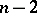, etc., and, finally, the-st polar is a straight line.

## Contents

How to Cite This Entry:
Polar. Encyclopedia of Mathematics. URL: http://encyclopediaofmath.org/index.php?title=Polar&oldid=14444
This article was adapted from an original article by A.B. Ivanov (originator), which appeared in Encyclopedia of Mathematics - ISBN 1402006098. See original article